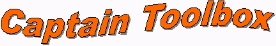### Time Variable Parameter (TVP) subset

The TVP functions form a subset of m-files from the Captain Toolbox for Matlab, allowing for the optimal estimation of time variable parameters in (dynamic) regression models: dynamic linear regression (dlr); dynamic harmonic regression (dhr); dynamic autoregression (dar); dynamic autoregression with exogenous variables (darx); and dynamic transfer function (dtf) models.

Fixed Interval Smoothing (FIS) estimates of the TVPs are obtained, under the assumption that the parameters vary as one of the family of generalised random walks: Random Walk (RW); Integrated Random Walk (IRW); Smoothed Random Walk (SRW); Local Linear Trend (LLT); and 1st order autoregression (AR(1)). In the case of dlr, dar, darx and dtf, the Noise Variance Ratio (NVR) and other hyper-parameters are optimised via Maximum Likelihood (ML) based on prediction error decomposition; while in the case of dhr, a special frequency domain optimisation is utilised, based on fitting the dhr model pseudo-spectrum to the logarithm of the AR spectrum. In comparison with most other algorithms for TVP estimation, the main innovations are the automatic hyper-parameter optimisation, the provision of FIS rather than the filtered TVP estimates and the special uses outlined below.

The Kalman Filtering and FIS algorithms are accessable via shells, primarily the functions dlr, dhr, dar, darx, dtf and univ. Such shells provide for ready estimation of the various special cases discussed below.

### Regression models

dhr: This is particularly useful for signal extraction and forecasting for periodic or quasi-periodic series. It provides smoothed estimates of the series, as well as all its components (trend, fundamental frequency and harmonic components), together with the estimated changing amplitude and phase of the latter. Typical applications are for the analysis of periodic environmental and economic time-series; restoration of noisy signals with gaps or other aberrations (e.g. audio); and evaluation of temporal changes in environmental data. (Example)

dlr: This can include the dhr, dar, darx and dtf as special cases but the regressors here are specified by the user. (Example)

### Stochastic dynamic models

dar: This is particularly useful for evaluating changing signal spectra and time-frequency analysis, since it provides the AR spectrum at each point in time based on the locally optimum time variable AR parameters. (Example)

darx: This is simply the extension of the DAR model to include measured exogeneous or input time series that are thought to affect the output.

dtf: This is an extension of the DARX model to allow for coloured noise in the output signal. The analysis employs Instrumental Variables (IV) to ensure that the parameter estimates are unbiased. Note that the Captain Input-Output modelling subset provides additional identification and estimation algorithms for such input-output analysis. The darx and dtf functions are included here as a link between the two Captain subsets.

sdp: This is an algorithm for state dependent parameter estimation, allowing for the non-parametric identification and forecasting of a very wide class of nonlinear systems, including chaotic processes.

### Trend + Autoregression models

univ: This is an alternative to the dhr analysis discussed above. Here, the trend is extracted from the time series and a peturbational component about the trend is modelled as a pure AR component. Such an approach is useful in cases where the periodic behaviour of the peturbation about the trend is not very marked.

### Selected references

A link to the abstract is provided for some papers.

• Ng, C.N. and Young, P.C., (1990), Recursive Estimation and Forecasting of Nonstationary Time Series, Journal of Forecasting, 9, 173-204.
• Young, P.C., (1988), Recursive extrapolation, interpolation and smoothing of nonstationary time series. In: H.F. Chen (ed.), Identification and System Parameter Estimation, Pergamon Press, Oxford, 33-44.
• Young, P.C., (1994), Time Variable Parameter and Trend Estimation in Nonstationary Economic Time Series, Journal of Forecasting, special issue on Time Series Advances in Economic Forecasting, 13, 179-210.
• Young, P., (1998), Data-based mechanistic modelling of Engineering Systems, Journal of Vibration and Control, 4, 5-28.
• Young, P., (1998), Data-based mechanistic modelling of environmental, ecological, economic and engineering systems, Environmental Modelling and Software, 13, 105-122.
• Young, P.C., (2000), Stochastic, dynamic modelling and signal processing, time variable and state dependent parameter estimation. Appears in Nonlinear and nonstationary signal processing, edited by W.J. Fitzgerald et al., Cambridge University Press, Cambridge, 74-114.
• Young, P.C. and Ng, C.N., (1989), Variance Intervention, Journal of Forecasting, 8, 399-416.
• Young, P.C. and Pedregal, D., (1999), Recursive and En-Bloc Approaches to Signal Extraction, Journal of Applied Statistics, 26, 103-128.
• Young, P.C., Ng, C.N. and Armitage, P., (1989), A Systems Approach to Economic Forecasting and Seasonal Adjustment. International Journal on Computers and Mathematics with Applications, special issue on System Theoretic Methods in Economic Modelling, 18, 481-501.c.taylor@lancaster.ac.uk 23-FEB-2011